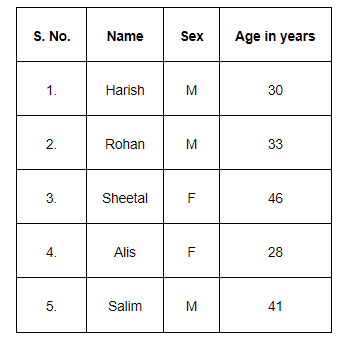# From the employees of a company, 5 persons are selected to represent them in the managing committee of the company.`
Question:

From the employees of a company, 5 persons are selected to represent them in the managing committee of the company. Particulars of five persons are as follows:A person is selected at random from this group to act as a spokesperson. What is the probability that the spokesperson will be either male or over 35 years?

Solution:

Let E be the event in which the spokesperson will be a male and F be the event in which the spokesperson will be over 35 years of age.

Accordingly, $\mathrm{P}(\mathrm{E})=\frac{3}{5}$ and $\mathrm{P}(\mathrm{F})=\frac{2}{5}$

Since there is only one male who is over 35 years of age,

$\mathrm{P}(\mathrm{E} \cap \mathrm{F})=\frac{1}{5}$

We know that $\mathrm{P}(\mathrm{E} \cup \mathrm{F})=\mathrm{P}(\mathrm{E})+\mathrm{P}(\mathrm{F})-\mathrm{P}(\mathrm{E} \cap \mathrm{F})$

$\therefore \mathrm{P}(\mathrm{E} \cup \mathrm{F})=\frac{3}{5}+\frac{2}{5}-\frac{1}{5}=\frac{4}{5}$

Thus, the probability that the spokesperson will either be a male or over 35 years of age is $\frac{4}{5}$.